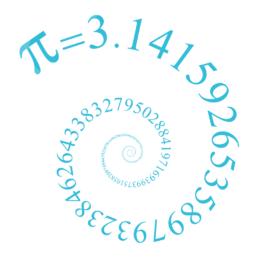Bournemouth Maths Tutor Barney M-T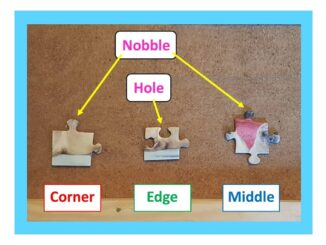HOW MUCH HARDER IS A 2000 PIECE JIGSAW THAN A 1000 PIECE JIGSAW?

First of all, it’s easy to see that a 2000 piece jigsaw is more than twice as hard as a 1000 piece jigsaw. To see why: imagine having two 1000 piece jigsaws that are of a similar style. Rather than complete them both separately (which would obviously be twice as hard as a 1000 pieceQuote of the week: DIFFERENTIATION IS A SKILL. INTEGRATION IS AN ART.

I was delighted to find this quote at the start of Seán M. Stewart‘s book “How to Integrate it“.One of the main differences between GCSE and A-level maths is the introduction of calculus. This branch of maths makes sense of the infinitely small, allowing us to deal with quantities that vary continuously (often over time)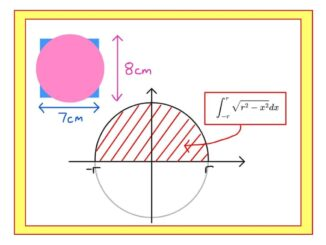WHAT’S THE POINT OF INTEGRATION BY SUBSTITUTION?

A PUZZLE: which of these shapes is larger: a square of side 7cm or a circle or diameter 8cm? Well that’s easy to answer if you know that the area of a circle is $A=\pi r^2$ but how do we know that $A=\pi r^2$ ?? Here is a particularly rigorous way that uses many of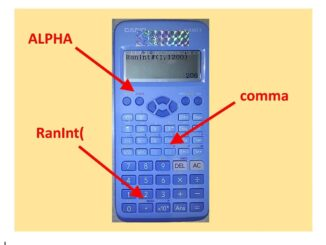TOP TEN SAMPLING METHODS

A quick reminder: if there are 1200 students at our school, it would be very time-consuming (if not impossible) to interview them all for your Sociology project. Here the population is everyone at the school, and interviewing them all would be called taking a census. Instead, it’s more practical to interview just a selection –PRODUCT OF PRIME FACTORS – A CALCULATOR SHORTCUT!

Expressing a positive integer (whole number) as the product of prime factors is a bit like dismantling a machine to see what it’s made of. Let’s try it with 26390. Drawing a Prime Factor Tree will take a while – but fortunately the calculator can do the hard work for us! Type in 26390, press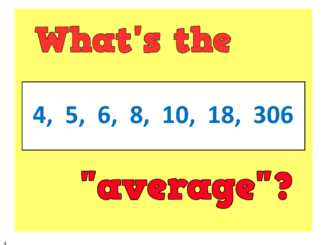WHAT IS THE “AVERAGE” IN MATHS?

An average is simply a way of picking out a typical value from a set of objects (usually numbers). There are infinitely many different ways to pick a “typical value”, but here are some of my favourites: Midrange average: The simplest of the lot: just calculate the number that is half way between the smallestHOW MANY SQUARES ARE THERE IN THIS GRID?

Before we see the quickest way to answer the question (scroll down if you are an expert!), let’s see some different possible approaches: GCSE APPROACH: There are 16 little squares.There is the one large square (the entire grid).But there are also some “2 by 2” squares, like the red one – I count 9 of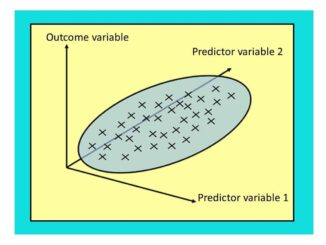Regression: lines (and planes) of best fit.

Let’s suppose we have some data for height and weight of some adult humans. We suspect the weight depends on the height – so taller people tend to weigh more than shorter. How can we develop this theory and use it to predict someone’s weight based on their height? Here’s a short introduction. AT GCSE:What does “MATH ERROR” mean?

SHORT ANSWER: this means you have just asked the calculator to do a sum it doesn’t like. The three most common reasons for getting this error message are: 1) you have tried to divide by zero: forbidden in maths! If you type in $3\div 0$ you are asking the calculator “zero times by what equals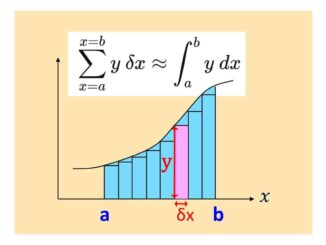WHAT’S ZERO TIMES BY INFINITY?

SHORT ANSWER: 0 x ∞ = anything you like! Intrigued? Read on… LONG ANSWER: the question seems absurd: after all, zero multiplied by anything is zero, yet any multiple of infinity is always infinity. But 0 x ∞ cannot be both zero and infinity, can it? Infinity doesn’t behave in the same way as other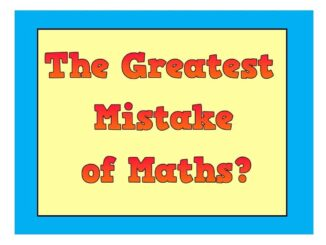THE GREATEST MISTAKE IN THE HISTORY OF MATHS:

This must surely be the exclusion of women from maths for most of our history, due to mathematics (and academia in general) being seen as an unsuitable activity or as simply “too hard” for women. As we see the female:male ratio among the best brains of mathematics now approaching 1:1, one wonders how much moreUSING MATHS TO CHECK WORLD RECORD ATTEMPTS: PETE’S DIABOLO HIGH THROW

My friend Pete can throw a diabolo ridiculously high – check out this video of him in action! Pete thinks his throw is higher than the “official” world record of 23.92metres so asked me if maths could measure his throw. So, how about it, maths? METHOD 1 (not recommended!): get a long tape measure and